# One Sample Z-Test Calculator

## Known standard deviation

Target: To check if the assumed μ0 is statistically correct, based on a sample average

Example1: A farmer calculated last year the average of the apples' weight in his apple orchard μ0 equals 17 kg, based on big sample
Current year he checked a small sample of apples and the sample average x equals 18 kg
Was the average of the apple's weight changed this year?

## Assumptions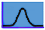Normal distributionThe standard deviations of the population is known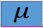Population expected mean is known

## Required Sample DataSample average of the population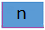Sample size of the population

## Hypotheses

H0: μ ≥ μ0 H0: μ = μ0 H0: μ ≤ μ0
H1: μ < μ0 H1: μ ≠ μ0 H1: μ > μ0

## Test statistic## Normal distribution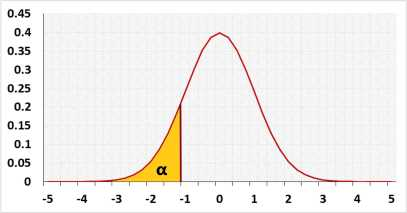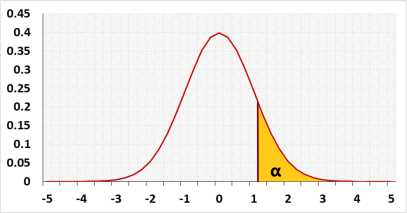## Test calculation

When enter raw data, the tool will run the Shapiro-Wilk normality test (when n≤5000) and calculate outliers, as part of the test calculation.
The test will validate the priori power

### Statistic data

 α: Significant level (0-1), maximum chance allowed rejecting H0 while H0 is correct (Type I Error) outliers: IncludedExcluded It is not recommended to exclude outliers unless you know the reasons effect ratio:effect size (unstandardized):standardized effect size: The power is expected to identify the effect. If one exists, H0 will be rejected. effect size - the change the test is expected to identifystandardized effect size - the change divided by the standard deviationeffect ratio - the effect size is a ratio from the expected mean
or or enter summarized data (x, n, σ, S) in following form

## Enter sample data

Header: You may change groups' name to the real names.
Data: When entering data, press Enter after each value

It is okay to leave empty cells, empty cells or non numeric cells won't be counted

## Sample data

You may copy data from Excel, Google sheets or any tool that separate data with Tab and Line Feed.
Copy the data, one column includes the header, and paste below.

It is okay to leave empty cells, empty cells or non numeric cells won't be counted

### Name:

 x: sample average μ0: expected population mean n: sample size σ: standard deviation S: sample standard deviation, S is not mandatory, but will help to validate σ skewness: normality p-val: shapiro wilk test outliers count: ,based on the Tukey's fences method, k=1.5

The difference between the expected SD and the sample SD

validation message